# K-theory

(diff) ← Older revision | Latest revision (diff) | Newer revision → (diff)

A part of algebraic topology that studies properties of vector bundles by algebraic and topological methods. As opposed to algebraic-theory, it is sometimes called topological-theory. In a wide sense, the term "K-theory" is used to denote the branch of mathematics that includes algebraic-theory and topological-theory, and it is characterized by specific algebraic and topological methods of investigation, which are called methods of-theory. In the narrow sense,-theory is the generalized cohomology theory (cf. Generalized cohomology theories) generated by the category of vector bundles (cf. Vector bundle).

The source of-theory is to be found in the skew-vector products (bundles) studied in algebraic topology, as well as in their numerous homotopic and algebraic properties. The most important properties of bundles and of concepts used in-theory are the properties of characteristic classes (cf. Characteristic class) of bundles, of classifying spaces (cf. Classifying space), of algebraic operations with bundles (direct sums, tensor products, exterior degrees), and of the inverse image of a bundle. A second source of-theory is the connection with algebraic-theory, which consists of the fact that the space of continuous sections of a vector bundle can be regarded as a module over the algebra of continuous functions, which turns out to be a projective module.

In analogy with-functors in algebraic-theory, the groupswere defined as the Grothendieck groups (cf. Grothendieck group) of the category of vector bundles withas base. Using the concept of an induced fibre bundle, the groups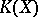are completed to define a functor from the category of topological spaces into the category of Abelian groups. Usually a-functor is studied not on the whole category of topological spaces, but on smaller subcategories. The category most often used is that of cellular spaces (complexes, cf. CW-complex). The definition of a-functor is extended to the category of pointed topological spaces and pairs of topological spaces and yields the groups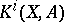,, by putting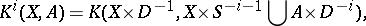where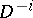is an-dimensional disc and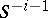is its boundary. The set of functors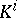,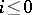, satisfies the axioms of generalized cohomology theory, which should be altered by taking into account the inequality.

One distinguishes the-theory built on the category of real vector bundles (real-theory) from the-theory built on complex vector bundles (complex-theory). Other variants of-theory are also studied, which take into account additional structures in certain bundles, for instance equivariant-theory.

A property of the groupwhich is important in the construction of generalized cohomology theory is Bott periodicity in complex-theory (cf. Bott periodicity theorem). It allows one, in particular, to get rid of the restrictionand to transform the functorsinto a-graded generalized cohomology theory. The fundamental importance of Bott periodicity is due to the vast computational possibilities of the constructed-theory.

The computational methods of generalized cohomology theory are applicable to-theory, among them, in particular, the method of spectral sequences (cf. Spectral sequence), allowing one to compute the groupsfor many classical finite-dimensional and infinite-dimensional spaces. For instance, if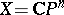is a complex projective space, thenwhere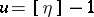, andis the one-dimensional canonical bundle over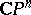.

For real-theory, a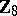-graded generalized cohomology theory is constructed using the Bott periodicity theorem. Applying supplementary algebraic structures in vector bundles, more general cohomology theories were constructed, organically containing complex, real, and symplectic-theories.

In the 1960s, with the help of-theory many problems both in topology and in other branches of mathematics were reconsidered. The most important results in-theory were connected with the systematic study of characteristic classes and of cohomology operations (cf. Cohomology operation) in terms of which theorems about the integral nature for multiplicative genera (analogues of the Riemann–Roch theorem) were proved, and simple and lucid solutions were given for classical problems connected with division algebras and with vector fields on spheres.

The methods of-theory were the origin for the development of many branches of topology, e.g. the theory of bordism. The proof of the Adams' conjecture on the description of the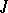-functor in terms of cohomology operations in-theory was of fundamental importance for the development of algebraic topology (see ).

Most impressive is the application of methods of-theory to the problem of computing the indices of elliptic operators. With the help of geometric constructions of vector bundles, far-reaching generalizations were obtained of the concepts of a differential and a pseudo-differential operator, of their symbols, of a Sobolev space, and of elliptic operators and their indices. Also, the Atiyah–Singer formulawas obtained ; it describes the index of an elliptic operator with symbolon a compact closed manifoldin terms of the Todd class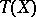and the Chern character of the operator. As corollaries to the Atiyah–Singer formula many special formulas have been obtained for various classes of operators which are most important in geometry, topology and other branches of mathematics. For instance, Hirzebruch's formula expresses the signature of an oriented compact, closed manifold in terms of the characteristic Pontryagin number of this manifold. The Hirzebruch formula itself and its generalizations to non-simply-connected manifolds is applied in differential topology to problems on the classification of smooth structures of manifolds.

In the 1970s generalizations of-theory connected with the applications in it of functional-analytic methods and with the adaptation of-theory to many problems of topology, geometry and of the theory of differential equations appeared. One of the generalizations consists of the replacement of the category of vector bundles by that of locally trivial fibre bundles (cf. Locally trivial fibre bundle) in which the fibres are finitely-generated modules which are projective over some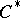-algebraand the structure groups of which are groups of automorphisms of these modules. Using this class of fibre bundles, non-trivial cohomology invariants were constructed for infinite-dimensional representations of infinite discrete groups. If a discrete groupis the fundamental group of a compact manifold admitting a Riemannian metric with non-positive two-dimensional curvature, then the characteristic numbers of the form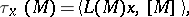that is, the higher signatures, are homology invariants. (Here,is an arbitrary rational Pontryagin–Hirzebruch class of the manifoldwith fundamental group.)

In a wider sense, the methods of-theory greatly influenced the development of ideas in differential topology. With the help of a combination of algebraic and topological-theories, problems in differential topology were solved about the classification of smooth and of piecewise-linear structures on manifolds, about the homological and topological invariance of the characteristic Pontryagin classes, etc. Methods of-theory have extensive applications in functional analysis, and in particular in the theory of Banach algebras (cf. Banach algebra).

How to Cite This Entry:
K-theory. Encyclopedia of Mathematics. URL: http://encyclopediaofmath.org/index.php?title=K-theory&oldid=12407
This article was adapted from an original article by A.S. Mishchenko (originator), which appeared in Encyclopedia of Mathematics - ISBN 1402006098. See original article